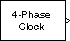# Multiphase Clock

Generate multiple binary clock signals

•Libraries:
DSP System Toolbox / Signal Management / Switches and Counters
DSP System Toolbox / Sources

## Description

The Multiphase Clock block generates a 1-by-N vector of clock signals, where you specify the integer N in the Number of phases parameter. Each of the N phases has the same frequency, f, specified in hertz by the Clock frequency parameter.

The clock signal indexed by the Starting phase parameter is the first to become active, at t=0. The other signals in the output vector become active in turn, each one lagging the preceding signal's activation by 1/(Nf) seconds, the phase interval. The period of the output is therefore 1/(Nf) seconds.

The active level can be either high (`1`) or low (`0`), as specified by the Active level (polarity) parameter. You specify the duration of the active level, D, as an integer between 1 and N-1 using the Number of phase intervals over which the clock is active parameter. This value specifies the number of phase intervals that each signal remains in the active state after becoming active. The active duty cycle of the signal is D/N.

## Ports

### Output

expand all

1-by-N vector of clock signals, where you specify N using the Number of phases parameter. For more information, see Description.

Data Types: `single` | `double` | `Boolean`

## Parameters

expand all

The frequency of all output clock signals, specified as a positive scalar.

The number of different phases, N, in the output vector, specified as a positive integer scalar.

The vector index of the output signal to first become active, specified as a scalar integer from `1` to N.

The duration of the active level, D, for every output signal specified as a scalar integer from 1 to N-1. The value you specify determines the number of phase intervals that each signal remains in the active state after becoming active. The active duty cycle of the signal is D/N.

The active level of the output, specified as ```High (1)``` or `Low (0)`.

The output data type, specified as `Logical` or `Boolean`.

## Block Characteristics

 Data Types `double` | `single` | `Boolean` Multidimensional Signals `No` Variable-Size Signals `No`

## Version History

Introduced before R2006a